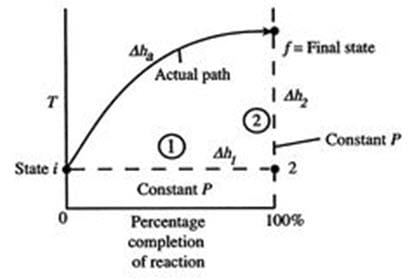# # ESTIMATION OF SOLID PROPELLANT ADIABATIC FLAME TEMPERATURE:

1. What Is Adiabatic Flame Temperature?
2. Estimation Of Solid Propellant Adiabatic Flame Temperature

# What Is Adiabatic Flame Temperature?

Consider a given combustion process that takes place adiabatically and with no work or changes in kinetic or potential energy involved. For such a process, the temperature of the products is referred to as the adiabatic flame temperature. With the assumptions of no work and no changes in kinetic or potential energy, this is the maximum temperature that can be achieved for the given reactants because any heat transfer from the reacting substances and any incomplete combustion would tend to lower the temperature of the products.

The maximum adiabatic flame temperature for a given fuel and oxidizer combination occurs with a stoichiometric mixture (correct proportions such that all fuel and all oxidizer are consumed). The amount of excess air can be tailored as part of the design to control the adiabatic flame temperature. The considerable distance between present temperatures in a gas turbine engine and the maximum adiabatic flame temperature at stoichiometric conditions, based on a compressor exit temperature of 1200°F(922 K).

## Estimation Of Solid Propellant Adiabatic Flame Temperature:

An initial view of the concept of adiabatic flame temperature is provided by examining two reacting gases, at a given pressure, and asking what the end temperature is. The process is shown schematically in the below figure, where temperature is plotted versus the percentage completion of the reaction. The initial state is  “i and the final state is “f” , with the final state at a higher temperature than the initial state. The solid line in the figure shows a representation of the “actual” process.Simplified Schematic Diagram Of Adiabatic Flame Temperature

To see how we would arrive at the final completion state the dashed lines break the state of reaction change into two parts. Process (1) is the reaction at constant T and P. To carry out such a process, we would need to extract heat. Suppose the total amount of heat extracted per unit mass is q1. The relation between the enthalpy changes in Process (1) is,

where q1 is the “heat of reaction”.

For Process (2), we put this amount back into the products to raise their temperature to the final level. For this process, The solid line in the figure shows a representation of the “actual” process.

or, if we can approximate the specific heat as constant (using some appropriate average value),

For the overall process, there is no work done and no heat exchanged so that the difference in enthalpy between initial and final states is zero:

The temperature change during this second process is therefore given by (approximately),

The value of the adiabatic flame temperature given in the above equation is for 100% completion of the reaction. In reality, as the temperature increases, the tendency is for the degree of reaction to be less than 100%. For example, for the combustion of hydrogen and oxygen, at high temperatures the combustion product (water) dissociates back into the simpler elemental reactants. The degree of reaction is thus itself a function of temperature that needs to be computed.

We used this idea in discussing the stoichiometric ramjet, when we said that the maximum temperature was independent of flight Mach number and hence of inlet stagnation temperature. It is also to be emphasized that the idea of a constant (average) specific heat, cp,avg , is for illustration and not inherently part of the definition of adiabatic flame temperature.

To find more topics on our website…